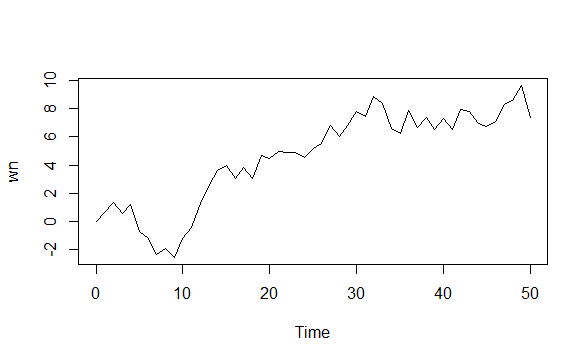# What is a Random Walk model and how can you simulate it using R?

A random walk is a simple example of non-stationary process.

A random walk has:

• No specified mean or variance
• Strong dependence over time
• It’s changes or increments are white noise

Simulating random walk in R:

``````arima.sim(model=list(order=c(0,1,0)),n=50)->rw
``````
``````ts.plot(rw)
``````